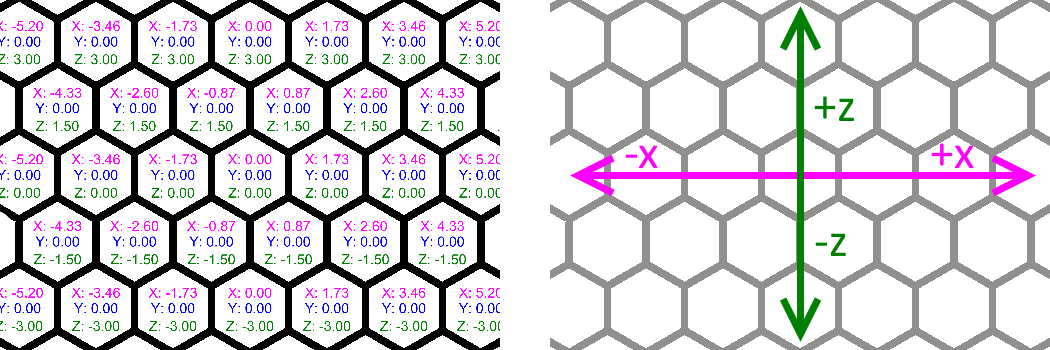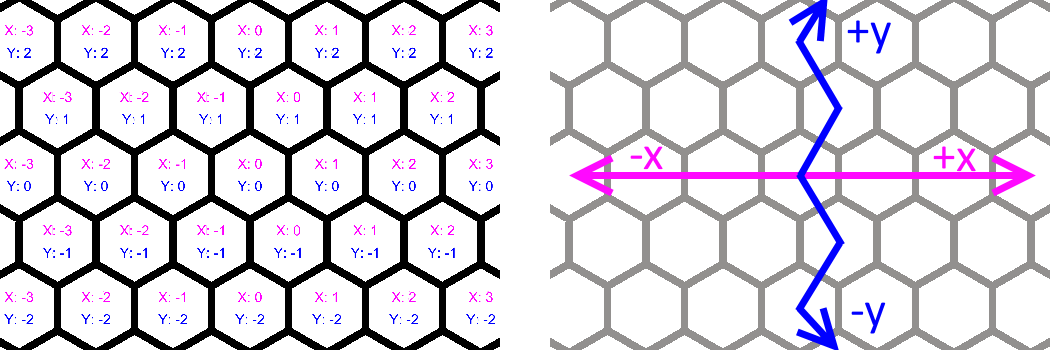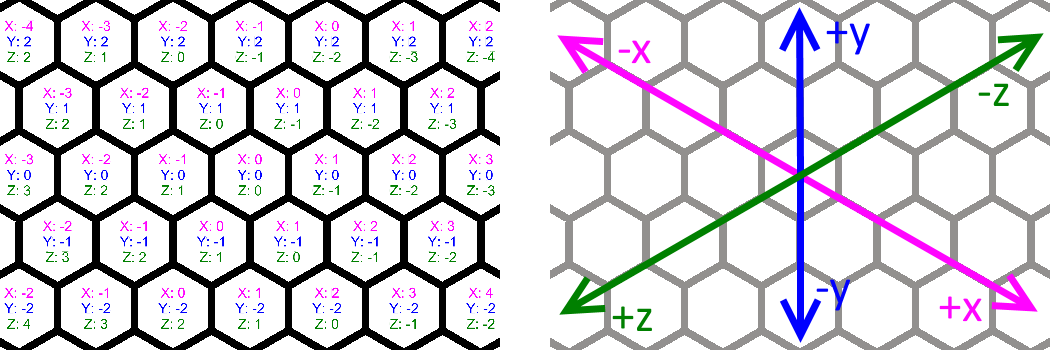HexMapLibrary
Wunderwunsch.HexMapLibrary.HexConverter Class Reference

Converts between cartesian coordinates and two different hexagonal-grid-coordinates (called "offset" and "cube") More...

## Static Public Member Functions

static Vector2Int CartesianCoordToOffsetCoord (Vector3 cartesianCoord)
converts the input cartesian coordinate to its equivalent offset tile coordinate More...

static Vector3Int CartesianCoordToTileCoord (Vector3 cartesianCoord)
converts the input cartesian coordinate to its equivalent tile coordinate More...

static Vector3 OffsetTileCoordToCartesianCoord (Vector2Int offsetTileCoord)
convers the input offset tile coordinate to its equivalent cartesian coordinate More...

static Vector3Int OffsetTileCoordToTileCoord (Vector2Int offsetTileCoord)
converts the input offset tile coordinate to its equivalent tile coordinate More...

static Vector3 TileCoordToCartesianCoord (Vector3Int tileCoord, float yCoord=0)
converts the input tile tile coordinate to its equivalent cartesian coordinate More...

static Vector2Int TileCoordToOffsetTileCoord (Vector3Int tileCoord)
converts the input tile coordinate to its equivalent offset coordinate More...

static Vector3 EdgeCoordToCartesianCoord (Vector3Int edgeCoord, float yCoord=0)
returns the midpoint of the edge in cartesian coordinates. More...

static Vector3Int CartesianCoordToClosestEdgeCoord (Vector3 cartesianCoord)
converts the input cartesian coordinate to the edge coordinate closest to it. More...

static Vector3Int CartesianCoordToClosestCornerCoord (Vector3 cartesianCoord)
converts the input cartesian coordinate to the cord coordinate closest to it. More...

static Vector3 CornerCoordToCartesianCoord (Vector3Int cornerCoord, float yCoord=0)
returns the cartesian coordinate of the input corner coordinate. More...

static Vector2 TileCoordToNormalizedPosition (Vector3Int tileCoordinate, float minX, float maxX, float minZ, float maxZ)

static Vector2 EdgeCoordToNormalizedPosition (Vector3Int edgeCoordinate, float minX, float maxX, float minZ, float maxZ)

static Vector2 CornerCoordToNormalizedPosition (Vector3Int cornerCoordinate, float minX, float maxX, float minZ, float maxZ)

static Vector2 CartesianCoordToNormalizedPosition (Vector3 cartesianCoordinate, float minX, float maxX, float minZ, float maxZ)

## Static Private Attributes

static float sqrt3 = Mathf.Sqrt(3)
cached value of square root of 3. More...

## Detailed Description

Converts between cartesian coordinates and two different hexagonal-grid-coordinates (called "offset" and "cube")

Using the cartesian coordinate system (the "normal" one you use all the time) does not work well with hexagons as you end up with fractional numbers which make things very non-intuitive and which are also often not suited to calculate on the grid. Therefore it is useful to use other coordinate systems which allow us to work with integer values and which are better suited to perform grid-based calculations.
This library uses two different hexagonal coordinate systems called "offset" and "cube", we mostly use the cube coordinate system as this is a lot easier to work with and has proper straight axes.
For most tasks you can just use the library without worrying too much about the details of the coordinate systems, however if you are interested to dive a bit deeper, I recommend reading Amit Patel's great introduction to hexagons: https://www.redblobgames.com/grids/hexagons/ .Coordinates & axes of cartesian coordinate systemCoordinates & 'axes' of offset coordinate systemCoordinates & axes of cube coordinate system

## ◆ CartesianCoordToOffsetCoord()

 static Vector2Int Wunderwunsch.HexMapLibrary.HexConverter.CartesianCoordToOffsetCoord ( Vector3 cartesianCoord )
static

converts the input cartesian coordinate to its equivalent offset tile coordinate

## ◆ CartesianCoordToTileCoord()

 static Vector3Int Wunderwunsch.HexMapLibrary.HexConverter.CartesianCoordToTileCoord ( Vector3 cartesianCoord )
static

converts the input cartesian coordinate to its equivalent tile coordinate

## ◆ OffsetTileCoordToCartesianCoord()

 static Vector3 Wunderwunsch.HexMapLibrary.HexConverter.OffsetTileCoordToCartesianCoord ( Vector2Int offsetTileCoord )
static

convers the input offset tile coordinate to its equivalent cartesian coordinate

## ◆ OffsetTileCoordToTileCoord()

 static Vector3Int Wunderwunsch.HexMapLibrary.HexConverter.OffsetTileCoordToTileCoord ( Vector2Int offsetTileCoord )
static

converts the input offset tile coordinate to its equivalent tile coordinate

## ◆ TileCoordToCartesianCoord()

 static Vector3 Wunderwunsch.HexMapLibrary.HexConverter.TileCoordToCartesianCoord ( Vector3Int tileCoord, float yCoord = `0` )
static

converts the input tile tile coordinate to its equivalent cartesian coordinate

Parameters
 tileCoord input tile coordinate yCoord explicitly sets cartesian y-coordinate

## ◆ TileCoordToOffsetTileCoord()

 static Vector2Int Wunderwunsch.HexMapLibrary.HexConverter.TileCoordToOffsetTileCoord ( Vector3Int tileCoord )
static

converts the input tile coordinate to its equivalent offset coordinate

## ◆ EdgeCoordToCartesianCoord()

 static Vector3 Wunderwunsch.HexMapLibrary.HexConverter.EdgeCoordToCartesianCoord ( Vector3Int edgeCoord, float yCoord = `0` )
static

returns the midpoint of the edge in cartesian coordinates.

## ◆ CartesianCoordToClosestEdgeCoord()

 static Vector3Int Wunderwunsch.HexMapLibrary.HexConverter.CartesianCoordToClosestEdgeCoord ( Vector3 cartesianCoord )
static

converts the input cartesian coordinate to the edge coordinate closest to it.

//TODO: is a bit inefficient and allocates memory, so improve later

## ◆ CartesianCoordToClosestCornerCoord()

 static Vector3Int Wunderwunsch.HexMapLibrary.HexConverter.CartesianCoordToClosestCornerCoord ( Vector3 cartesianCoord )
static

converts the input cartesian coordinate to the cord coordinate closest to it.

//TODO: is a bit inefficient and allocates memory, so improve later

Parameters
 cartesianCoord
Returns

## ◆ CornerCoordToCartesianCoord()

 static Vector3 Wunderwunsch.HexMapLibrary.HexConverter.CornerCoordToCartesianCoord ( Vector3Int cornerCoord, float yCoord = `0` )
static

returns the cartesian coordinate of the input corner coordinate.

## ◆ sqrt3

 float Wunderwunsch.HexMapLibrary.HexConverter.sqrt3 = Mathf.Sqrt(3)
staticprivate

cached value of square root of 3.